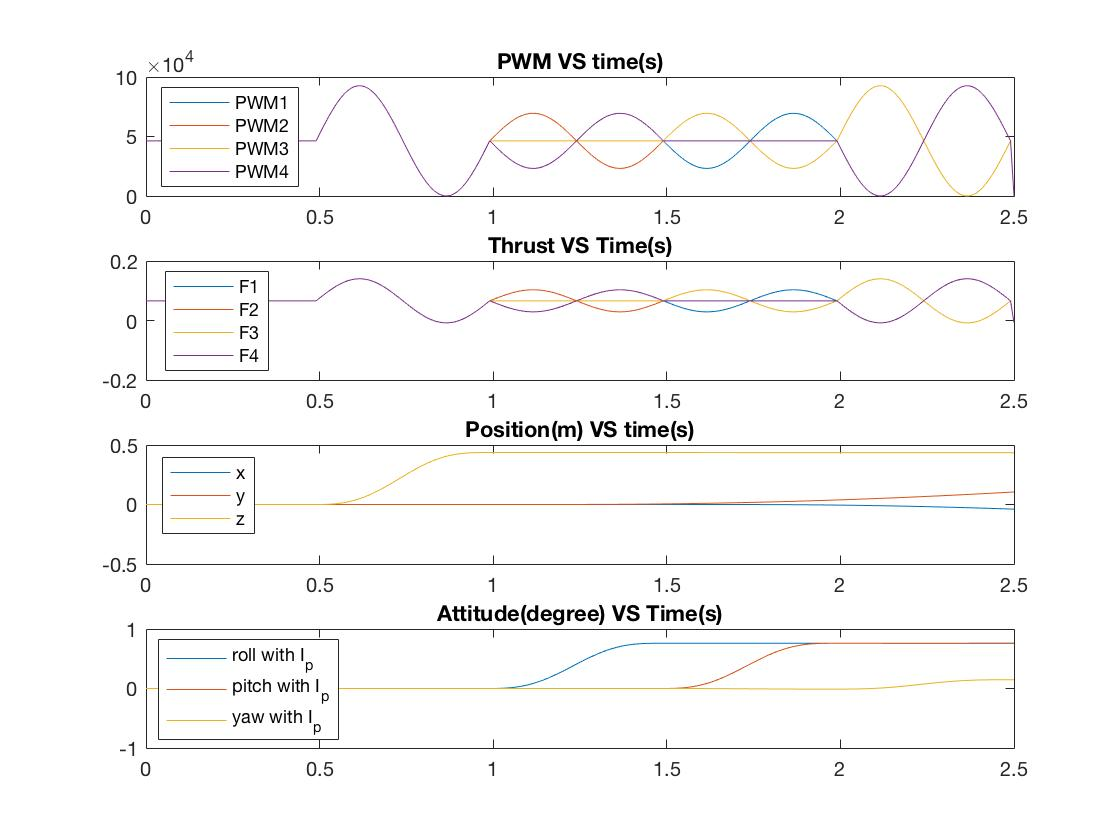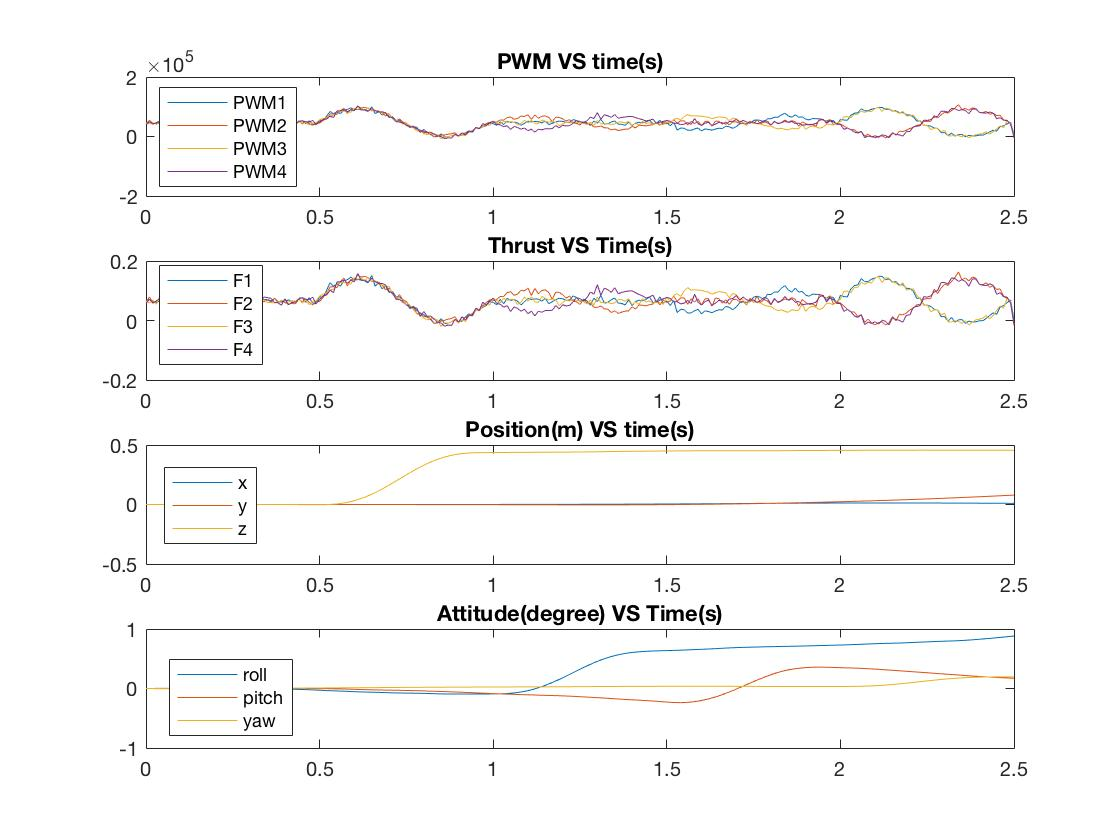20 Nov

# Four Motor Drone Dynamic model simulations

The first simulation is performed with an ideal drone model. The PWMs for each motor were changed over time to achieve hover, z motion and roll, pitch and yaw motions. No noise were introduced in this simulation. Figure 1 shows the result of this simulation. For t = 0 ~ 0.5 s, PWMs were set to identical, constant values so that the total thrust is equal to mg. From the simulation result, drone remains hovering.

• For t = 0.5 ~ 1 s, all PWMs changed together, which results to a z direction motion.
• For t = 1 ~ 1.5 s, PWM2 and PWM4 were changed and leads to roll motion.
• For t = 1.5 ~ 2 s, PWM1 and PWM3 were changed and leads to pitch motion.
• For t = 2 ~ 2.5 s, PWM1 and PWM3 were changed together, PWM2 and PWM4 were changed together. This leads to yaw motion.###### Figure 1 - Matlab simulation of ideal four motor drone

In figure 2, gussian noise were added to the PWMs. The simulation results shows the position and attitude of the system changed when noise presents.